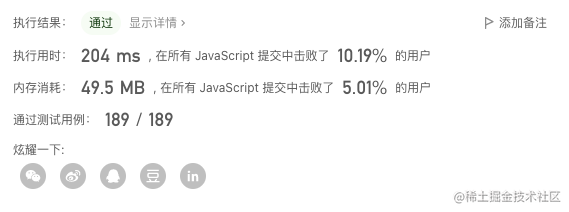dev-zuo 技术日常

# 编程思维训练 - 递归、滑动窗口、动态规划

## 两数之和

``````输入：nums = [2,7,11,15], target = 9

``````

``````输入：nums = [3,2,4], target = 6

``````

``````输入：nums = [3,3], target = 6

``````

``````2 <= nums.length <= 104
-109 <= nums[i] <= 109
-109 <= target <= 109

``````

### 普通解法 O(n²)

• 普通解法思路：使用两层循环遍历出所有 a，b 可能的场景，逐一计算比较，找到 target 值，返回对应 index 数组
• 时间复杂度: O(n²)
``````var twoSum = function (nums, target) {
let len = nums.length;
for (let i = 0; i < len - 1; i++) {
for (let j = i + 1; j < len; j++) {
if (nums[i] + nums[j] === target) {
return [i, j]
}
}
}
}
``````

### 一层循环解法 O(n)

• 优化思路：一层循环遍历时，a、b 会经历两次遍历，这是突破口。增加一个对象，存储之前已经遍历过的值的下标 obj[nums[index]] = index。如果有发现当前遍历项 obj[target - nums[index]] 不为 undefined，就说明找到匹配项了。一层遍历就可以解决
• 时间复杂度：O(n)
``````var twoSum = function (nums, target) {
let len = nums.length;
let obj = {} // js 直接用对象代替 hash 表
for (let i = 0; i < len; i++) {
if (obj[target - nums[i]] !== undefined) {
return [i, obj[target - nums[i]]]
}
obj[nums[i]] = i
}
}
``````

## 斐波那契数

``````F(0) = 0，F(1) = 1
F(n) = F(n - 1) + F(n - 2)，其中 n > 1
``````

``````输入：n = 2

``````

``````输入：n = 3

``````

``````输入：n = 4

``````

### 递归解法

``````var fib = function(n) {
if (n <= 1) {
return n
}
return fib(n - 1) + fib(n - 2)
}
``````

### 记忆化递归解法

``````let cache = {}
var fib = function(n) {
if (n <= 1) {
return n
}
if (cache[n]) {
return cache[n]
}
let result = fib(n - 1) + fib(n - 2)
cache[n] = result
return result
}
``````

### 递归栈溢出问题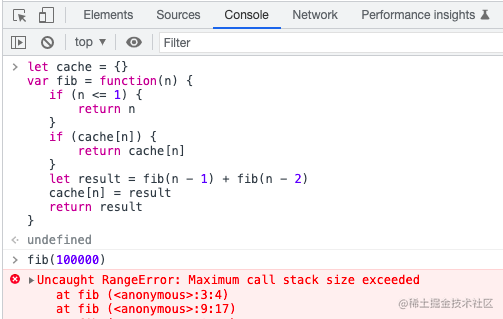### 动态规划解法

``````var fib = function(n) {
let dp = [0, 1]
if (n <= 1) {
return dp[n]
}
for (let i = 2, len = n; i <= n; i++) {
dp[i] = dp[i - 1] + dp[i - 2]
}
return dp[n]
}
``````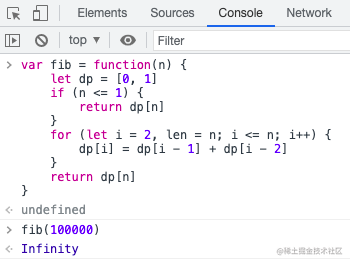### 节省空间 DP

``````var fib = function(n) {
if (n <= 1) {
return n
}
let pre1 = 0, pre2 = 1, result = 0;
for (let i = 2; i <= n; i++) {
result = pre1 + pre2
pre1 = pre2
pre2 = result
}
return result
}
``````

### 矩阵快速幂与通项公式

1. 矩阵快速幂 - 复杂度 O(logn) 递推关系：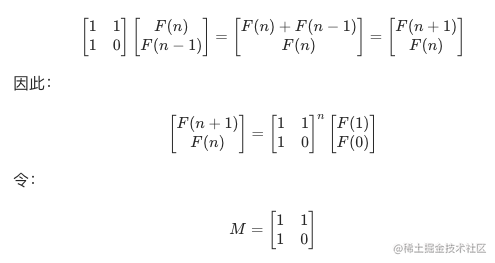``````// 来源：力扣官方题解
var fib = function(n) {
if (n < 2) {
return n;
}
const q = [[1, 1], [1, 0]];
const res = pow(q, n - 1);
return res;
};

const pow = (a, n) => {
let ret = [[1, 0], [0, 1]];
while (n > 0) {
if ((n & 1) === 1) {
ret = multiply(ret, a);
}
n >>= 1;
a = multiply(a, a);
}
return ret;
}

const multiply = (a, b) => {
const c = new Array(2).fill(0).map(() => new Array(2).fill(0));
for (let i = 0; i < 2; i++) {
for (let j = 0; j < 2; j++) {
c[i][j] = a[i] * b[j] + a[i] * b[j];
}
}
return c;
}
``````
1. 通项公式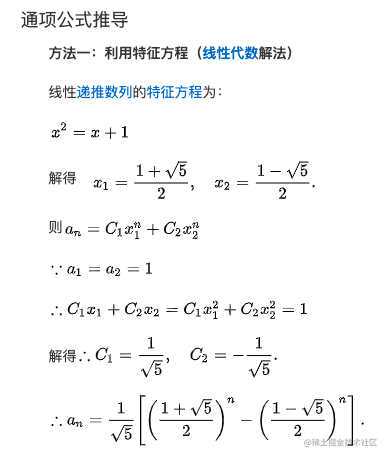``````// 通项公式看 Math.pow 性能， 缺点 fib(20) 6765.000000000005，浮点计算不准确，可 Math.round(result)
// 公式推导：https://baike.baidu.com/item/斐波那契数列/99145
var fib = function(n) {
return (Math.pow((1 + Math.pow(5, 0.5)) / 2, n) - Math.pow((1 - Math.pow(5, 0.5)) / 2, n)) /  Math.pow(5, 0.5)
}
``````

### 爬楼梯问题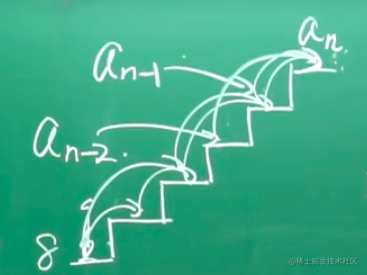1. 从 n-1 台阶爬一个台阶，这种情况爬到 n，总共有 sum(n-1) 种方法
2. 从 n-2 台阶爬两个台阶，这种情况爬到 n，总共有 sum(n-2) 种方法

### 猴子跳阶梯问题

``````1. 一天一只顽猴想要从山脚爬到山顶
2. 途中经过一个有n个台阶的阶梯，但是这个猴子有个习惯，每一次只跳1步或3步
3. 试问猴子通过这个阶梯有多少种不同的跳跃方式
``````

## 递归与地图

### 扫雷

``````// 部分核心代码，c语言实现
int mark = {{0,1},{1,1},{1,0},{1,-1},{0,-1},{-1,-1},{-1,0},{-1,1}}; // 8个方向坐标
char map[NUM][NUM] = {0}; // 内部地图
void zero_digui(int x, int y) // 递归显示
{
ui[x-1][y-1] = 0 + 48;
int x1 = 0, y1 = 0;
for (int i = 0; i < 8; i++) { // 遍历周围的8个方向
x1 = x + mark[i];
y1 = y + mark[i];
if (x1 < 1 || x1 > NUM || y1 < 1 || y1 > NUM) continue; // 如果超出了边界
int count = lei_count(x1,y1,NUM); // 计算当前坐标周围有多少雷
if (count != 0) ui[x1-1][y1-1] = count + 48; // 不为0直接写值
else if (ui[x1-1][y1-1] == '0') continue; // 为0, 但值已是0，以前遍历过
else zero_digui(x1,y1); // 为0，没遍历过, 遍历
}
}
``````

### 机器人走迷宫

1. 房间由 X*Y 的方格组成，6*4 的大小。每一个方格以坐标（x,y）描述。
2. 机器人固定从方格（0，0）出发，只能向东或者向北前进。出口固定为房间的最东北角，如下图的方格（5，3）.用力保证机器人可以从入口走到出口。
3. 房间有些方格是墙壁，如（4，1），机器人不能经过哪儿。
4. 有些地方是一旦到达就无法走到出口的，如标记为B的方格，称之为陷阱方格。有些地方是机器人无法到达的，如标记为A的方格，称之为不可达方格，不可达方格不包括墙壁所在的位置。
5. 如图中 陷阱方格有 2 个 不可达方格有 3 个。
6. 请为该机器人实现路径规划功能：给定房间大小，墙壁位置，请计算出陷阱方格与不可达方格分别有多少个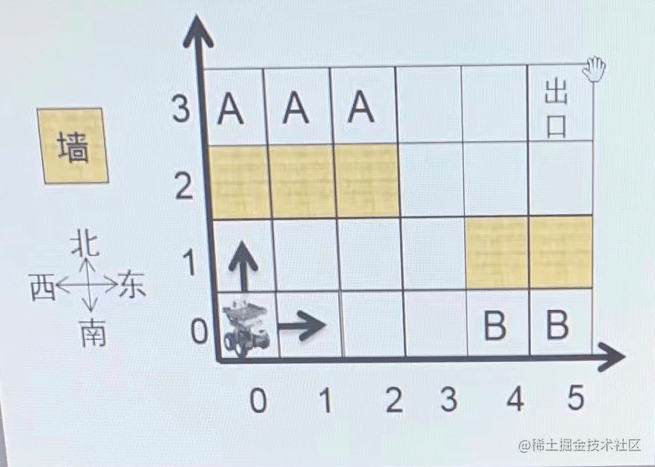1. 第一行为房间的 X 跟 Y `（0<X,Y<=1000）`
2. 第二行为房间中的墙壁的个数 N `（0<=N<X*Y）`
3. 接着下面会有N行墙壁的坐标同一行中如果有多个数据以一个空格隔开，用例保证所有数据合法（结尾不带回车换行）

1. 陷阱方格与不可达方格数量，两个信息在一行中输出，以一个空格隔开

``````# 输入
6 4   # 房间 x, y
5     # 墙壁个数
0 2   # 墙壁坐标
1 2
2 2
4 1
5 1

# 输出
2 3
``````

• 和爬楼梯类似，使用逆向思维，从终点倒推，可以到达终点的点。依次递归标记（可到达终点）并计数，递归完成（坐标都越界）后，就可以计算出陷阱（不能到达终点的点个数）方格个数；
• 同样的方法，正向递归标记，就可以计算出不可达（不能从起点到达的点）方格数量。
• 这里递归有点特殊，向上、向右两个方向都需要递归，注意退出条件
``````function getTrapAndUnreachable(x, y, wallList) {
// 生成 x * y 二维数组，并设置墙
const getMap = () => {
let mapList = new Array(x)
for (let i = 0; i < x; i++) {
mapList[i] = new Array(y).fill('')
}
wallList.forEach(([x, y])=> {
// console.log(x, y)
mapList[x][y] = 'wall'
})
console.log(mapList)
return mapList
}

let mapList = getMap()
let reachable = 0
// 从终点遍历，能够到达终点的点
const backSearchFill = (x, y) => {
mapList[x][y] = 'reachable' // 必定可达
reachable++ // reachable 数++
// x, y 可到达终点，则左(x - 1, y)、下(x, y - 1)都可达
// console.log(x, y,[x - 1, y], [x, y - 1])
if (x - 1 < 0 && y - 1 < 0) {
return // 如果左下两个坐标不满足条件，直接退出
}
// 左侧查找, 如果左边(x - 1, y)没超出坐标范围，且不是墙, 标记可达，并继续向下递归寻找
if (x - 1 >= 0 && !['wall', 'reachable'].includes(mapList[x - 1][y])) {
backSearchFill(x - 1, y)
}
// 下查找（根据上面的规律：在范围内，不是墙）
if (y - 1 >= 0 && !['wall', 'reachable'].includes(mapList[x][y-1])) {
backSearchFill(x, y - 1)
} else {
return
}
}
backSearchFill(x - 1, y - 1)
let trapCount = x * y - reachable - wallList.length
console.log(mapList, `陷阱（不能到达终点的点个数）：\${trapCount}`)

// 重新初始化
mapList = getMap()
reachable = 0
let maxX = x - 1;
let maxY = y - 1;
// 从起点遍历，能够从起点到达的点
const frontSearchFill = (x, y) =>  {
mapList[x][y] = 'reachable' // 必定可达
reachable++ // reachable 数++
// x, y 能够从起点达到，则右(x + 1, y)、上(x, y + 1)都能从起点到达
// console.log(x, y,[x + 1, y], [x, y + 1])
if (x + 1 > maxX && y + 1 > maxY) {
return // 如果左下两个坐标不满足条件，直接退出
}
// 右侧查找, 如果右边(x + 1, y)没超出坐标范围，且不是墙, 且没标记为可达，并继续向下递归寻找
if (x + 1 <= maxX && !['wall', 'reachable'].includes(mapList[x + 1][y])) {
frontSearchFill(x + 1, y)
}
// 上查找（根据上面的规律：在范围内，不是墙）
if (y + 1 <= maxY && !['wall', 'reachable'].includes(mapList[x][y + 1])) {
frontSearchFill(x, y + 1)
} else {
return
}
}
frontSearchFill(0, 0)
let unreachableCount = x * y - reachable - wallList.length
console.log(mapList, `不可达（不能从起点到达的点）：\${unreachableCount}`)

console.log(trapCount, unreachableCount)
}

console.log(getTrapAndUnreachable(6, 4, [[0, 2], [1, 2], [2,2], [4,1], [5,1]]))
// [
//     [ '', '', 'wall', '' ],
//     [ '', '', 'wall', '' ],
//     [ '', '', 'wall', '' ],
//     [ '', '', '', '' ],
//     [ '', 'wall', '', '' ],
//     [ '', 'wall', '', '' ]
//   ]
//   [
//     [ 'reachable', 'reachable', 'wall', 'reachable' ],
//     [ 'reachable', 'reachable', 'wall', 'reachable' ],
//     [ 'reachable', 'reachable', 'wall', 'reachable' ],
//     [ 'reachable', 'reachable', 'reachable', 'reachable' ],
//     [ '', 'wall', 'reachable', 'reachable' ],
//     [ '', 'wall', 'reachable', 'reachable' ]
//   ] 陷阱（不能到达终点的点个数）：2
//   [
//     [ '', '', 'wall', '' ],
//     [ '', '', 'wall', '' ],
//     [ '', '', 'wall', '' ],
//     [ '', '', '', '' ],
//     [ '', 'wall', '', '' ],
//     [ '', 'wall', '', '' ]
//   ]
//   [
//     [ 'reachable', 'reachable', 'wall', '' ],
//     [ 'reachable', 'reachable', 'wall', '' ],
//     [ 'reachable', 'reachable', 'wall', '' ],
//     [ 'reachable', 'reachable', 'reachable', 'reachable' ],
//     [ 'reachable', 'wall', 'reachable', 'reachable' ],
//     [ 'reachable', 'wall', 'reachable', 'reachable' ]
//   ] 不可达（不能从起点到达的点）：3
//   2 3
``````

## 无重复字符的最长子串

``````输入: s = "abcabcbb"

``````

``````输入: s = "bbbbb"

``````

``````输入: s = "pwwkew"

请注意，你的答案必须是 子串 的长度，"pwke" 是一个子序列，不是子串。
``````

``````0 <= s.length <= 5 * 104
``````

s 由英文字母、数字、符号和空格组成

### 暴力穷举法 - pass 99% 超时

``````// 986 / 987 个通过测试用例，最后一个超时
var lengthOfLongestSubstring = function (s) {
if (s.length <= 1) {
return s.length
}
let maxLen = 0;
const hasRepeat = (str) => {
let charCountObj = {}
for (let i = 0, len = str.length; i < len; i++) {
let item = str[i]
if (charCountObj[item]) {
return true
} else {
charCountObj[item] = 1
}
}
return false
}
for (let i = 0; i < s.length; i++) {
for (let j = i + 1; j <= s.length; j++) {
let str = s.substring(i, j + 1);
if (maxLen >= str.length || hasRepeat(str)) {
continue
}
maxLen = str.length
}
}
return maxLen
};
``````

### 穷举加缓存 - pass 99% 超出内存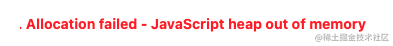``````// 986 / 987 个通过测试用例 - 超过内容限制
var lengthOfLongestSubstring = function (s) {
if (s.length <= 1) {
return s.length
}
let maxLen = 0;
let cacheHasRepeat = {} // 缓存之前已经判重后的结果
const hasRepeat = (str) => {
// 如果有缓存直接使用缓存结果，减少重复遍历
if (cacheHasRepeat[str] !== undefined) {
return cacheHasRepeat[str]
}
let charCountObj = {}
for (let i = 0, len = str.length; i < len; i++) {
let item = str[i]
if (charCountObj[item]) {
cacheHasRepeat[str] = true // 缓存结果
return true
} else {
charCountObj[item] = 1
}
}
cacheHasRepeat[str] = false // 缓存结果
return false
}
for (let i = 0; i < s.length; i++) {
for (let j = i + 1; j <= s.length; j++) {
let str = s.substring(i, j + 1);
if (maxLen >= str.length || hasRepeat(str)) {
continue
}
maxLen = str.length
}
}
return maxLen
};
``````

### 滑动窗口解法 - AC耗时500ms

"abcabcbb"

...

``````var lengthOfLongestSubstring = function (s) {
// 滑动窗口解法
if (s.length <= 1) {
return s.length
}
let longest = 0
// (a)bcabcbb
for (let i = 0, len = s.length; i < len; i++) {
let charObj = { [s[i]]: 1 } // 存储字符出现次数 { a : 1 }
let max = 1
for (let j = i + 1; j < s.length; j++) {
if (charObj[s[j]]) { // 有值，说明重复了，跳出循环
break;
} else {
// 没重复的，编辑字符出现次数为 1
charObj[s[j]] = 1
max++
}
}
(max > longest) && (longest = max)
}
return longest
}
``````

### 优化后的滑动窗口 80ms

``````var lengthOfLongestSubstring = function(s) {
if (s.length <= 1) {
return s.length
}
let longest = 0
let charSet = new Set()
let rk = 1 // 右指针 index
for (let i = 0, len = s.length; i < len; i++) {
// 第一次遍历，规规矩矩
if (i === 0) {
} else {
// 利用上一次结果, 开启下一次判断
// 假设第二次，从 "b" 开始,  把上次循环重复的 "a" 移除
// 第二次 rk 还是上次的 5，不用从 b 的后一位重复判断
charSet.delete(s[i - 1])
}

// 没走到字符串末尾，且没有重复的
while(rk < len && !charSet.has(s.charAt(rk))) {
// 添加到无重复集合
// 右指针偏移 +1
rk++
}
// i = 0; "a - e"，rk 为最后一个 "a" 的值，5,5 - 0 为 5
((rk - i) > longest) && (longest = rk - i)
}
return longest
};
``````

## 零钱兑换

``````输入：coins = [1, 2, 5], amount = 11

``````

``````输入：coins = , amount = 3

``````

``````输入：coins = , amount = 0

``````

```````1 <= coins.length <= 12`
`1 <= coins[i] <= 231 - 1`
`0 <= amount <= 104`
``````

### 穷举所有情况

1. 全为 1
2. 全为 2
3. 全为 5
4. 1 2 组合， 个数比例遍历
5. 1 5 组合， 个数比例遍历
6. 2 5 组合，个数比例遍历
7. 1 2 5 组合， 个数比例遍历

15=1×11+4×1，硬币数 5 个

15=3×5，硬币数 3 个

### 取巧的递归方法

``````# 金额11,硬币数0 => for循环依次减去硬币面额,硬币数+1 => 继续减,硬币+1
f(11, 0) => -1 f(10, 1) => -1 f(9, 2) => .. f(x, 3)
=> -2 f(8, 2)
=> -5 f(5, 2)
=> -2 f(9, 1) => -1 f(8, 2)
=> -2 f(7, 2)
=> -5 f(4, 2)
=> -5 f(6, 1) => -1 f(5, 2)
=> -2 f(4, 2) => for(1,2,5),硬币+1...
=> -5 f(1, 2) => -1 f(0, 3) // ok比最小值
=> -2 f(-1, 3) 退出循环
``````

``````var coinChange = function(coins, amount) {
if (amount === 0) {
return 0
}
let min = Infinity
const f = (sum, count) => {
// 为负数时，表示走不通
if (sum < 0) {
return
}
// 可以拼凑成功
if (sum === 0) {
count < min && (min = count)
return count
}
for (let i = 0, len = coins.length; i < len; i++) {
f(sum - coins[i], count+1)
}
}
f(amount, 0)
// 如果找不到
if (min === Infinity) {
return -1
}
return min
};
``````

``````15 / 189 个通过测试用例
[1,2,5] 100 超出时间限制
``````

### 动态规划 DP

``````# f(金额) 为 凑成金额所需的最小硬币数
f(0) = 0
# f(金额) = 1 + 其中硬币最小值 (f(金额-1), f(金额-2), f(金额-5))
f(1) = 消耗 1 硬币 + Min(f(0), f(-1), f(-4)）= 1 + min(0 ,-1,-1)
f(2) = 消耗 1 硬币 + Min(f(1), f(0), f(-3)) = 1 + min(1, 0, -1)
f(3) = 消耗 1 硬币 + Min(f(2), f(1), f(-2)) = 1 + min(1, 1, -1)
f(4) = 消耗 1 硬币 + Min(f(3), f(2), f(-1)) = 1 + min(2, 1, -1)
f(5) = 消耗 1 硬币 + Min(f(4), f(3), f(0)) = ...
f(6) = 消耗 1 硬币 + Min(f(5), f(4), f(1)) = ...
...
f(11) = 消耗 1 硬币 + Min(f(10), f(8), f(6))
``````

``````var coinChange = function(coins, amount) {
if (amount === 0) {
return 0
}
let dp = 
// dp(1) => dp(2) => dp(3) => ... dp(n)
for (let i = 1; i <= amount; i++) {
let min = Infinity
// 遍历所有减去 1 个硬币后的值，找最小值， -1 忽略
for (let j = 0, len = coins.length; j < len; j++) {
let count = dp[i - coins[j]]
// 负数时直接忽略
if (count < 0) {
continue
}
// 0 或 其他值
if (count < min) {
min = count
}
}
// 如果是 Infinity，则为 -1
if (min === Infinity) {
dp[i] = -1
} else {
dp[i] = min + 1
}
}
return dp[amount]
};
``````